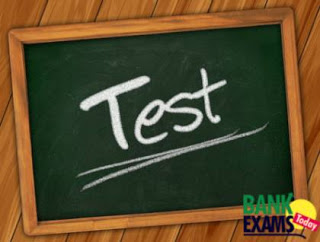# Quant Test for IBPS PO Pre Part - 17#### Ques 1.

After receiving two successive raises,Ravi’s salary become equal to 15/8 times of his initial salary. By how much percent was the salary raised the first time if the second raise was twice as high (in percent) as the first?

Solution :
Lets Initial salary = X
First raised salary % = Y
Second raised salary % = 2Y
As per problem
==> X *  (100+Y)/100  * (100+2Y)/100  = 15/8 * X
==> (100+Y)*(100+2Y) = 15/8 * 100 *100
==> 2Y^2 + 300Y - 8750 = 0
==> Y = 25

#### Ques 2.

A Merchant gives 3 consecutive discounts of 10%, 15% and 15% after which he sells his goods at a percentage profit of 30.05% on the C.P. Find the value of the percentage profit that the shopkeeper would have earned if he had given discount of 10% and 15% only.

Solution :
Assume Marked Priced = 100
After 3 discounts selling price = 100(90/100)*(85/100)*(85/100) = 65.025
Still he getting 30.05 % profit
Cost Price = (65.025*100)/130.05 = 50
If he Allows only 10% and 15% discount Selling Price = 100 *(90/100) *(85/100) = 76.5
Then he gets % of profit = (26.5/50) *100 = 53%

#### Ques 3.

A sum of Rs. 1000 after 3 years at compound interest becomes a certain amount that is equal to the amount that is the result of a 3 year depreciation from Rs. 1728. Find the difference between the rates of CI and depreciation. (GivenCI is 10% p.a.).

Solution :
Amount after 3 years = 1000(1+(10/100)^3 = 1331
Depreciation Rate = X%
1728*(1-(X/100))^3 = 1331
==> X = 100/12
Difference = 10 - (100/12) = 20/12 =5/3%

#### Ques 4.

A mixture can be prepared by sugar and salt. Price of sugar is thrice the price of salt. Siva sells the mixture at Rs. 4320 per 20g, thereby making a profit of 20%. If the ratio of sugar and salt in the mixture be 2 : 3, find the cost price of sugar.

Solution :
Sugar = 3X
Salt = X
Selling Price of 1 kg = 4320/20 = 216
Cost Price of 1 kg = 216 *100/120 = 180

3X                                 X

180

180-X                 3X-180

==> (180-X)/(3X-180)  = 2/3
==> X = 100
Sugar = 3*100 = 300

#### Ques 5.

The probability that a contractor will get a plumbing contract is 2/3 and the probability that he will get an electric contract is 5/9. If the probability of getting at least one contract is 4/5, what is the probability that he will get both the contracts?

Solution :
Probability of getting At least one  Contract = 4/5
Probability of getting both contracts = X
==>2/3 + 5/9 -x = 4/5
==> X = 19/45

#### Ques 6.

In an examination it is required to get 900 marks of the aggregate marks to pass. One of the students got 43% marks and he was declared failed by 40 marks. Find the maximum aggregate marks of the examination.

Solution :
As per problem
==> (43X/100) + 40 = 900
==> X = 2000

#### Ques 7.

P, Q and R invested Rs 10000, Rs 12000 and Rs 1300 in a business. After the end of 4 months all of them withdrew Rs 1000. After a total of 8 months from the start of business all of them added Rs 1000 in their investments. Find the ratio of their shares in total profit at the end of a year.

Solution :
Ratio =5000*8 + 4500*4 : 6000*8 + 5500*4 : 6500*8 + 6000*4
==>50*2 + 45 : 60*2 + 55 : 65*2 + 60
==>10*2 + 9 : 12*2 + 11 : 13*2 + 12
==>29 : 35 : 38

#### Ques 8.

Two circles have their circumferences equal to 440 m and 528 m respectively. What is the difference between the area of the larger circle and the smaller circle?

Solution :
Circle 1  circumferences = 440
==> 2*pi *R1 = 440
==> R1 = 70
Circle 2 circumferences = 528
==> 2*pi*R2 = 528
==> R2 = 84
Difference between Areas = Pi*R2^2 - Pi*R1^2 = 22/7 (84+70)(84-70)
==> Difference areas = 6776

#### Ques 9.

9000   1795   355   68   ?   1.32

Solution :
9000 / 5 – 5 = 1795
1795 / 5 – 4 = 355
355 / 5 – 3 = 68
68 / 5 – 2 = 11.6

#### Ques 10.

x^3=10648    ;  y^2=484

Solution :
x^3 =10648
==>x = 22

y^2= 484
==>y = + 22, – 22
X ≥ Y